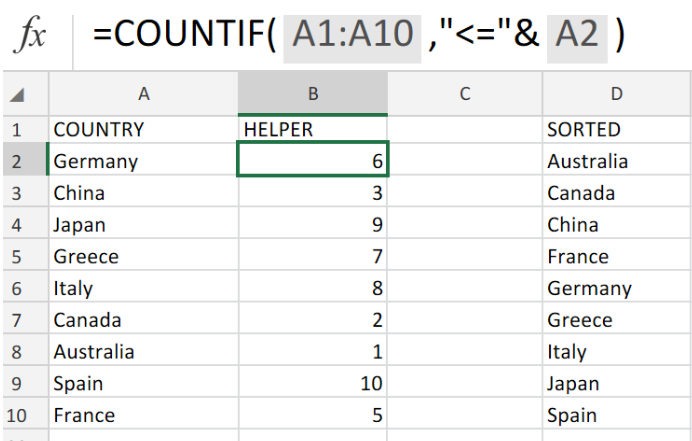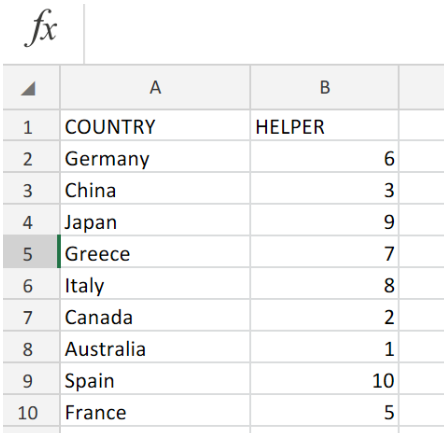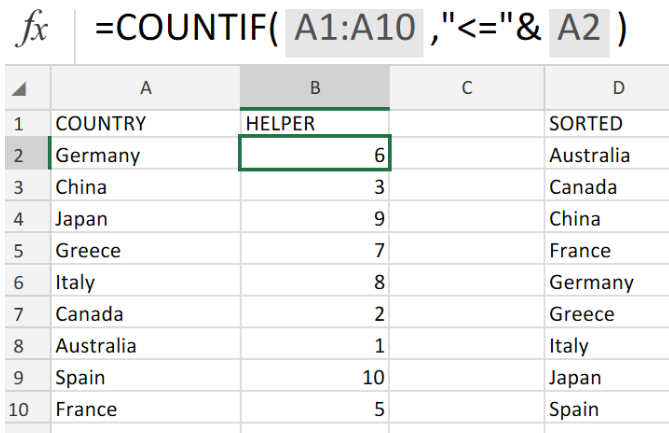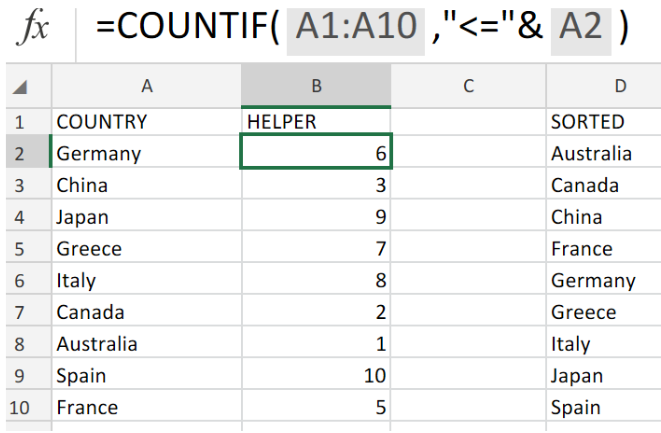Get instant live expert help with Excel or Google Sheets“My Excelchat expert helped me in less than 20 minutes, saving me what would have been 5 hours of work!”

#### Post your problem and you'll get expert help in seconds

Your message must be at least 40 characters
Our professional experts are available now. Your privacy is guaranteed.

# What is the Basic Text Sort Formula in Excel?Figure 1. of Basic Text Sort Formula in Excel.

In order to alphabetically sort text values in Excel, we can utilize the COUNTIF Function. This tutorial will assist all levels of Excel users in sorting text values.

Generic Formula

`=COUNTIF(range,"<="&A1)`

When the text is compared by Excel, it will automatically rank them by  deciding which of the values provided is greater.

## How to use the COUNTIF Function in Excel.

In the example illustrated below, we are going to utilize the COUNTIF Function to rank the listed countries in alphabetical order..

We can achieve this by following two simple steps.

1. We must first list the COUNTRIES in one column. We will label the next column HELPER where the COUNTIF Function will determine the number value of each country. .

We can use the HELPER column to store the sorted values according to their rank.Figure 2. of  Text Data for Sorting in Excel.

1. The COUNTIF formula syntax which we will input in cell B2 of the example illustrated above, is as follows;

`=COUNTIF(A1:A10,"<="&A2)`Figure 3. of the COUNTIF Function in Excel.

Copy the operation syntax in cell B2 down the HELPER column to achieve complete results.

## Note

In the example illustrated above;

• COUNTRIES = cell range A1:A10
• HELPER = B2:B10

The COUNTIF formula syntax works by using the greater than or equal to (>=) symbol operator together with text.Figure 4. of Final Result.

## Instant Connection to an Expert through our Excelchat Service:

Our live Excelchat Service is here for you. We have Excel Experts available 24/7 to answer any Excel questions you may have. Guaranteed connection within 30 seconds and a customized solution for you within 20 minutes

### Did this post not answer your question? Get a solution from connecting with the expert.Another blog reader asked this question today on Excelchat:
Related blogs## Subscribe to Excelchat.coAnother blog reader asked this question today on Excelchat: